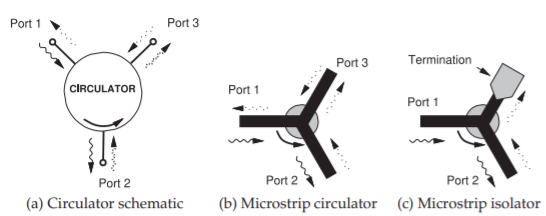# 11.13: Ferrite Components- Circulators and Isolators

$$\newcommand{\vecs}{\overset { \rightharpoonup} {\mathbf{#1}} }$$ $$\newcommand{\vecd}{\overset{-\!-\!\rightharpoonup}{\vphantom{a}\smash {#1}}}$$$$\newcommand{\id}{\mathrm{id}}$$ $$\newcommand{\Span}{\mathrm{span}}$$ $$\newcommand{\kernel}{\mathrm{null}\,}$$ $$\newcommand{\range}{\mathrm{range}\,}$$ $$\newcommand{\RealPart}{\mathrm{Re}}$$ $$\newcommand{\ImaginaryPart}{\mathrm{Im}}$$ $$\newcommand{\Argument}{\mathrm{Arg}}$$ $$\newcommand{\norm}{\| #1 \|}$$ $$\newcommand{\inner}{\langle #1, #2 \rangle}$$ $$\newcommand{\Span}{\mathrm{span}}$$ $$\newcommand{\id}{\mathrm{id}}$$ $$\newcommand{\Span}{\mathrm{span}}$$ $$\newcommand{\kernel}{\mathrm{null}\,}$$ $$\newcommand{\range}{\mathrm{range}\,}$$ $$\newcommand{\RealPart}{\mathrm{Re}}$$ $$\newcommand{\ImaginaryPart}{\mathrm{Im}}$$ $$\newcommand{\Argument}{\mathrm{Arg}}$$ $$\newcommand{\norm}{\| #1 \|}$$ $$\newcommand{\inner}{\langle #1, #2 \rangle}$$ $$\newcommand{\Span}{\mathrm{span}}$$$$\newcommand{\AA}{\unicode[.8,0]{x212B}}$$

Circulators and isolators are nonreciprocal devices that preferentially route microwave signals. The essential element is a disc (puck) of ferrite which when magnetized supports a preferred direction of propagation due to the gyromagnetic effect.

## 11.8.1 Gyromagnetic Effect

An electron has a quantum mechanical property called spin (there is not a spinning electron) creating a magnetic moment $$m$$, see Figure $$\PageIndex{1}$$(a). In most materials the electron spin occurs in pairs with the magnetic moment of one electron canceled by the oppositely-directed magnetic moment of an other electron. However, in some materials the spin does not occur in pairs and there is a net magnetic moment.

A most interesting microwave property occurs when a magnetic material is biased by a DC magnetic field resulting in the gyromagnetic effect. This affects the way an RF field propagates and this is described by a nine element permeability called a tensor. The permeability of a magnetically biased magnetic material is:

$\label{eq:1}\left[\mu\right]=\left[\begin{array}{ccc}{\mu_{xx}}&{\mu_{xy}}&{\mu_{xz}}\\{\mu_{yx}}&{\mu_{yy}}&{\mu_{yz}}\\{\mu_{zx}}&{\mu_{zy}}&{\mu_{zz}}\end{array}\right]=\left[\begin{array}{ccc}{\mu_{0}}&{0}&{0}\\{0}&{\mu}&{\jmath\kappa}\\{0}&{-\jmath\kappa}&{\mu}\end{array}\right]$

That is,

$\label{eq:2}\left[\begin{array}{c}{B_{x}}\\{B_{y}}\\{B_{z}}\end{array}\right]=\left[\begin{array}{ccc}{\mu_{0}}&{0}&{0}\\{0}&{\mu}&{\jmath\kappa}\\{0}&{-\jmath\kappa}&{\mu}\end{array}\right]\left[\begin{array}{c}{H_{x}}\\{H_{y}}\\{H_{z}}\end{array}\right]$

Thus a $$z$$-directed $$H$$ field produces a $$y$$-directed $$B$$ field. The effect on propagation of an EM field is shown in Figure $$\PageIndex{1}$$(b). The EM wave does not travel in a straight line and instead curves, in this case, to the right. Thus forward- and backward-traveling waves diverge from each other and propagation is not reciprocal. This separates forward- and backward-traveling waves.

## 11.8.2 Circulator

A circulator exploits the gyromagnetic effect. Referring to the schematic of a circulator shown in Figure $$\PageIndex{2}$$(a), where the arrows indicate that the signal that enters Port $$1$$ of the circulator leaves the circulator at Port $$2$$ and not at Port $$3$$. Similarly power that enters at Port $$2$$ is routed to Port $$3$$, andFigure $$\PageIndex{1}$$: Magnetic moments: (a) electron spin; and (b) Gyromagnetic effect impact on the propagation of EM waves in a magnetic material with an externally applied magnetic bias field.Figure $$\PageIndex{2}$$: Ferrite components with a magnetized ferrite puck in the center in (b) and (c).

power entering at Port $$3$$ is routed to Port $$1$$. In terms of $$S$$ parameters, an ideal circulator has the scattering matrix

$\label{eq:3}\mathbf{S}=\left[\begin{array}{ccc}{0}&{0}&{S_{13}}\\{S_{21}}&{0}&{0}\\{0}&{S_{32}}&{0}\end{array}\right]=\left[\begin{array}{ccc}{0}&{0}&{1}\\{1}&{0}&{0}\\{0}&{1}&{0}\end{array}\right]$

A microstrip circulator is shown in Figure $$\PageIndex{2}$$(b), where a disc of magnetized ferrite can be placed on top of a microstrip Y junction to realize a preferential direction of propagation of the EM fields. In the absence of the biasing magnetic field, the circulation function does not occur.

## 11.8.3 Circulator Isolation

The isolation of a circulator is the insertion loss from what is the output port to the input port, i.e. in the reverse direction. Referring to the circulator in Figure $$\PageIndex{2}$$(a), if port $$1$$ is the input port there are two output ports and so there are two isolations equal to the return loss from port $$3$$ to port $$2$$, and from port $$2$$ to port $$1$$. The smaller of these is the quoted isolation. If this is an ideal circulator and port $$2$$ is perfectly matched, then the isolation would be infinite. Then the $$S$$ parameters of the circulator are

$\label{eq:4}\mathbf{S}=\left[\begin{array}{ccc}{\Gamma}&{\alpha}&{T}\\{T}&{\Gamma}&{\alpha}\\{\alpha}&{T}&{\Gamma}\end{array}\right]\approx\left[\begin{array}{ccc}{0}&{0}&{1}\\{1}&{0}&{0}\\{0}&{1}&{0}\end{array}\right]$

where $$T$$ is the transmission factor, $$\Gamma$$ is the reflection coefficient at the ports, and $$\alpha$$ is the leakage.

## 11.8.4 Isolator

Isolators are devices that allow power flow in only one direction. Figures $$\PageIndex{2}$$(c) and $$\PageIndex{3}$$ show microstrip isolators based on three-port circulators. Power entering Port $$1$$ as a traveling wave is transferred to the ferrite and emerges at Port $$2$$. Virtually none of the power emerges at Port $$3$$. A traveling wave signal applied at Port $$2$$ appears at Port $$3$$, where it is absorbed in a termination created by resistive material placed on top of the microstrip. The resistive material forms a lossy transmission line and no power is reflected. Thus power can travel from Port $$1$$ to Port $$2$$, but not in the reverse direction.Figure $$\PageIndex{3}$$: A microstrip isolator operating from $$29$$ to $$31.5\text{ GHz}$$. Isolator in (b) has the dimensions $$5\text{ mm}\times 6\text{ mm}$$ and is $$6\text{ mm}$$ high. The isolator supports $$2\text{ W}$$ of forward and reverse power with an isolation of $$18\text{ dB}$$ and insertion loss of $$1\text{ dB}$$. Renaissance 2W9 series, copyright Renaissance Electronics Corporation, used with permission.Figure $$\PageIndex{4}$$: Frequency conversion using a mixer.

This page titled 11.13: Ferrite Components- Circulators and Isolators is shared under a CC BY-NC license and was authored, remixed, and/or curated by Michael Steer.